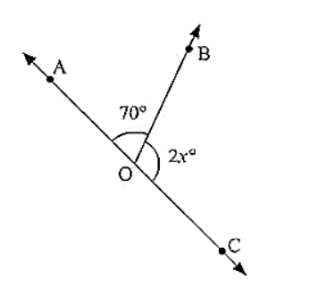# In the below figure, AOC is a line, find x.

Question:

In the below figure, AOC is a line, find x.Solution:

Since ∠AOB and ∠BOC are linear pairs,

∠AOB + ∠BOC = 180°

70 + 2x = 180

2x = 180 - 70

2x = 110

x = 110/2

x = 55

Hence, the value of x is 55°